Chapter 15

The conservation of energy is one of the most basic physics laws, for the electromagnetic field, the energy conservation equation is as follows: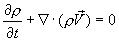(15.1)

In which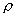is the mass density,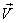is the field velocity.

The mass density and energy density have the following relationship: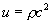In which u is the electromagnetic field energy density.

From equation (14.15) and (14.16), we have: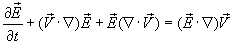(15.2)

And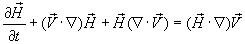(15.3)

Thus: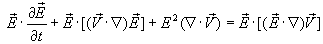(15.4)

And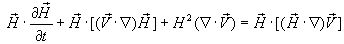(15.5)

Thus: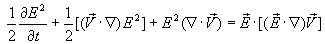(15.6)

And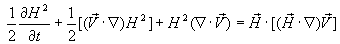(15.7)

Combine (15.6) and (15.7), thus: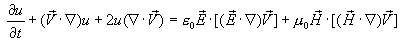(15.8)

Let us assume that the electromagnetic energy is conserved, therefore, we have: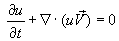(15.9)

Thus: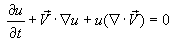(15.10)

Thus, we have: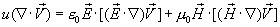(15.11)

As we know: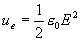(15.12)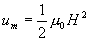(15.13)

Let us define: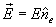(15.14)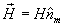(15.15)

From the electromagnetic field energy conservation equation, then we can get the following equation: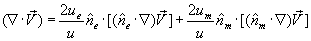(15.16)

Then we can rewrite the electromagnetic field as follows: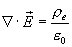(15.17)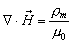(15.18)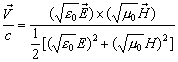(15.19)(15.20)(15.21)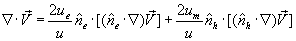(15.22)